# Lyapunov surfaces and curves

(diff) ← Older revision | Latest revision (diff) | Newer revision → (diff)

A class of surfaces and curves that have quite good smoothness properties; it was introduced in potential theory by A.M. Lyapunov at the turn into the 20th century.

A surfacein the three-dimensional Euclidean space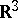is called a Lyapunov surface if it satisfies the following three conditions (Lyapunov's conditions): 1) at every point ofthere is a well-defined tangent plane, and consequently a well-defined normal; 2) there is a number, the same for all points of, such that if one takes the part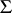oflying inside the Lyapunov sphere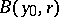with centre at an arbitrary point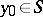and radius, then the lines parallel to the normal toat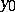meetat most once; and 3) there are two numbers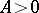and,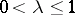, the same for the whole of, such that for any two points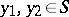,(*)

whereis the angle between the normals toat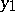and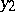. Sometimes these three conditions are supplemented by the requirement thatis closed and that the solid angle under which any partofis visible at an arbitrary point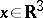is uniformly bounded.

The Lyapunov conditions can be generalized to hypersurfaces in,.

Similarly, a simple continuous curvein the plane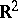is called a Lyapunov curve if it satisfies the following conditions:) at every point ofthere is a well-defined tangent, and consequently a well-defined normal; and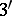) there are two numbersand,, the same for the whole of, such that for any two points(*) holds, whereis the angle between the tangents or normals toatand. Here Lyapunov's condition 2) follows from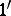) and). The Lyapunov curves are a subclass of the simple smooth curves.

How to Cite This Entry:
Lyapunov surfaces and curves. Encyclopedia of Mathematics. URL: http://encyclopediaofmath.org/index.php?title=Lyapunov_surfaces_and_curves&oldid=14936
This article was adapted from an original article by E.D. Solomentsev (originator), which appeared in Encyclopedia of Mathematics - ISBN 1402006098. See original article